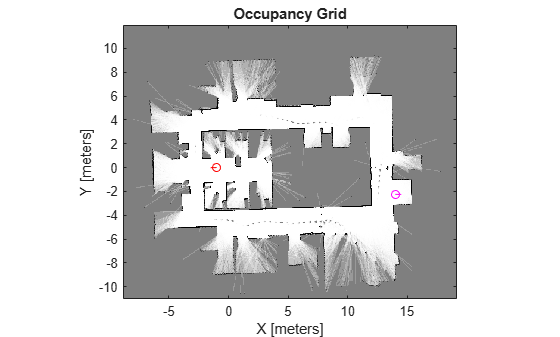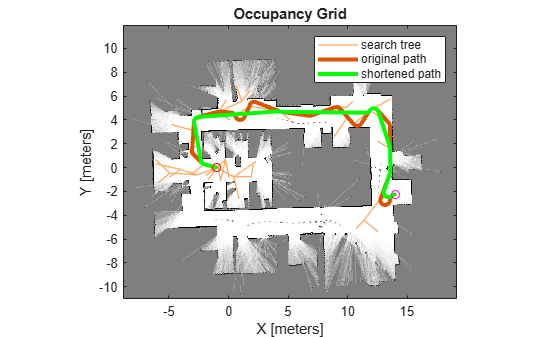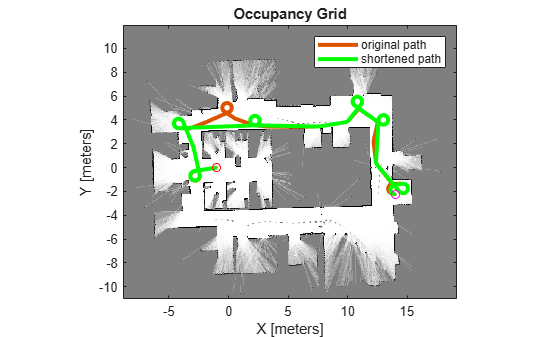# Plan Mobile Robot Paths Using RRT

This example shows how to use the rapidly exploring random tree (RRT) algorithm to plan a path for a vehicle through a known map. Special vehicle constraints are also applied with a custom state space. You can tune your own planner with custom state space and path validation objects for any navigation application.

Load an existing occupancy map of a small office space. Plot the start and goal poses of the vehicle on top of the map.

```load("office_area_gridmap.mat","occGrid") show(occGrid) % Set start and goal poses. start = [-1.0,0.0,-pi]; goal = [14,-2.25,0]; % Show start and goal positions of robot. hold on plot(start(1),start(2),'ro') plot(goal(1),goal(2),'mo') % Show start and goal headings. r = 0.5; plot([start(1),start(1) + r*cos(start(3))],[start(2),start(2) + r*sin(start(3))],'r-') plot([goal(1),goal(1) + r*cos(goal(3))],[goal(2),goal(2) + r*sin(goal(3))],'m-') hold off```### Define State Space

Specify the state space of the vehicle using a `stateSpaceDubins` object and specifying the state bounds. This object limits the sampled states to feasible Dubins curves for steering a vehicle within the state bounds. A turning radius of 0.4 meters allows for tight turns in this small environment.

```bounds = [occGrid.XWorldLimits; occGrid.YWorldLimits; [-pi pi]]; ss = stateSpaceDubins(bounds); ss.MinTurningRadius = 0.4;```

### Plan The Path

To plan a path, the RRT algorithm samples random states within the state space and attempts to connect a path. These states and connections need to be validated or excluded based on the map constraints. The vehicle must not collide with obstacles defined in the map.

Create a `validatorOccupancyMap` object with the specified state space. Set the `Map` property to the loaded `occupancyMap` object. Set a `ValdiationDistance` of 0.05 m. This validation distance discretizes the path connections and checks obstacles in the map based on this.

```stateValidator = validatorOccupancyMap(ss); stateValidator.Map = occGrid; stateValidator.ValidationDistance = 0.05;```

Create the path planner and increase the max connection distance to connect more states. Set the maximum number of iterations for sampling states.

```planner = plannerRRT(ss,stateValidator); planner.MaxConnectionDistance = 2.0; planner.MaxIterations = 30000;```

Customize the `GoalReached` function. This example helper function checks if a feasible path reaches the goal within a set threshold. The function returns `true` when the goal has been reached, and the planner stops.

`planner.GoalReachedFcn = @exampleHelperCheckIfGoal;`
```function isReached = exampleHelperCheckIfGoal(planner, goalState, newState) isReached = false; threshold = 0.1; if planner.StateSpace.distance(newState, goalState) < threshold isReached = true; end end ```

Plan the path between the start and goal. Reset the random number generator for reproducible results.

```rng default [pthObj, solnInfo] = plan(planner,start,goal);```

### Plot the Path

Show the occupancy map. Plot the search tree from the `solnInfo`. Interpolate and overlay the final path.

```show(occGrid) hold on % Plot entire search tree. plot(solnInfo.TreeData(:,1),solnInfo.TreeData(:,2),'.-'); % Interpolate and plot path. interpolate(pthObj,300) plot(pthObj.States(:,1),pthObj.States(:,2),'r-','LineWidth',2) % Show start and goal in grid map. plot(start(1),start(2),'ro') plot(goal(1),goal(2),'mo') hold off```### Customize Dubins Vehicle Constraints

To specify custom vehicle constraints, customize the state space object. This example uses `ExampleHelperStateSpaceOneSidedDubins`, which is based on the `stateSpaceDubins` class. This helper class limits the turning direction to either right or left based on a Boolean property, `GoLeft`. This property essentially disables path types of the `dubinsConnection` object.

Create the state space object using the example helper. Specify the same state bounds and give the new Boolean parameter as `true` (left turns only).

```% Only making left turns goLeft = true; % Create the state space ssCustom = ExampleHelperStateSpaceOneSidedDubins(bounds,goLeft); ssCustom.MinTurningRadius = 0.4;```

### Plan Path

Create a new planner object with the custom Dubins constraints and a validator based on those constraints. Specify the same `GoalReached` function.

```stateValidator2 = validatorOccupancyMap(ssCustom); stateValidator2.Map = occGrid; stateValidator2.ValidationDistance = 0.05; planner = plannerRRT(ssCustom,stateValidator2); planner.MaxConnectionDistance = 2.0; planner.MaxIterations = 30000; planner.GoalReachedFcn = @exampleHelperCheckIfGoal;```

Plan the path between the start and goal. Reset the random number generator again.

```rng default [pthObj2,solnInfo] = plan(planner,start,goal);```

### Plot Path

Draw the new path on the map. The path should only execute left turns to reach the goal.

```figure show(occGrid) hold on % Show the search tree. plot(solnInfo.TreeData(:,1),solnInfo.TreeData(:,2),'.-'); % Interpolate and plot path. pthObj2.interpolate(300) plot(pthObj2.States(:,1), pthObj2.States(:,2), 'r-', 'LineWidth', 2) % Show start and goal in grid map. plot(start(1), start(2), 'ro') plot(goal(1), goal(2), 'mo') hold off```